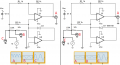# Determine AC impedance of the resistor using Arduino and precision full-wave rectifier

#### kostia_callentis

Joined Aug 2, 2023
1
Hello,

I'm trying to build a circuit that would allow measuring AC impedance (real part) using Arduino. I have a signal generator that generates a sine wave. I'm using full-wave precision rectifier to remove negative portion of the signal so it can be read by analogue input pin on the Arduino UNO. I use 2 resistors in series and knowing voltage input and voltage across unknow resistor, and also resistance of one of the resistors, I determine the resistance of an unknown resistor (I call it resistance for simplicity, the frequency is adjusted to minimize the reactance).
This method works when I do not try to remove negative portion of the signal read across the unknown resistor, but once I hoop up a precision rectifier in parallel to the unknown resistor it starts working improperly. Please see the images of the circuits attached. On the left oscilloscope "A" shows how the given rectifier works, "C" shows the input voltage and "B" shows the voltage drop across the unknown resistor. In the right image I need to remove negative voltage from the "B" to read it but the output signal "A" and also "B" become like in a half-wave rectifier and the magnitudes of the signal also do not represent a proper drop in voltage due to an unknown resistor.
Could somebody help me understand what I'm doing wrong here? Thank you!#### Ian0

Joined Aug 7, 2020
8,938
The input resistance of your circuit is 1k and it is asymmetrical. The output impedance of your potential divider is 33k.
You are not dividing the input signal by 3 (50k/150k), but on one half of the waveform you are dividing it by 1k/100k.
The impedance of your measuring circuit must be so high that it does not affect the circuit it is measuring.
Use another op-amp as a buffer at point B to drive your rectifier.

By the way, you don't say how you intend to measure the rectified circuit with the arduino.